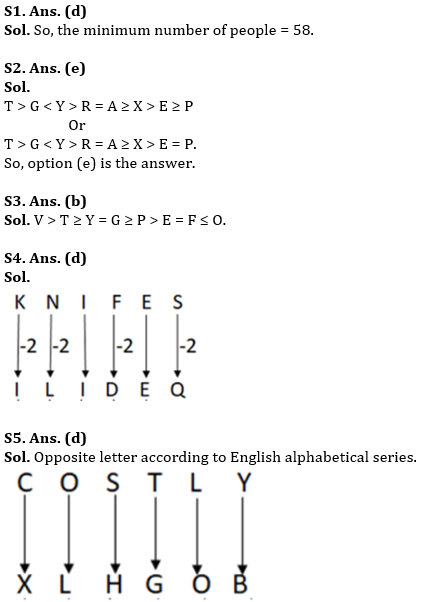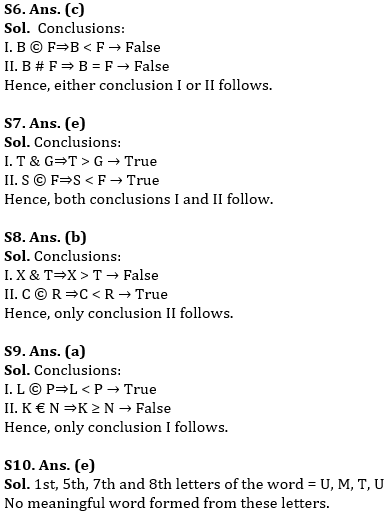Latest Banking jobs   »   sbi po reasoning

# Reasoning Quiz For SBI PO Prelims 2023 -14th September

Q1. In a dinner party, a certain number of people sit in a row and facing towards North direction, Madhav is 29th from the left corner and Shantanu is 32nd from the right corner. If only Girish sits exactly between them. Then, what could be the minimum number of people in the party?
(a) 62
(b) 37
(c) 48
(d) 58
(e) None of the above

Q2. Which of the following symbols should replace the blank in the given expression in order to make the expression A > P definitely true?
T > G < Y > R = A ≥ X > E _ P
(a) ≤
(b) ≥
(c) <
(d) =
(e) Either (b) or (d)

Q3. Which of the following symbols should replace the blank in the given expression in order to make the expression P ≤ T definitely true?
V > T ≥ Y = G _ P > E = F ≤ O
(a) ≤
(b) ≥
(c) <
(d) >
(e) None of these

Q4. In a certain code language, if ‘TRACES’ is coded as ‘RPAAEQ’ and ‘CLUSTER’ is coded as ‘AJUQREP’, then what is the code for ‘KNIFES’?
(a) ILIDFQ
(b) ILICEQ
(c) ILIDER
(d) ILIDEQ
(e) None of these

Q5. In a certain code language, if ‘LAPTOP’ is coded as ‘OZKGLK’ and ‘APPLE’ is coded as ‘ZKKOV’, then what is the code for ‘COSTLY’?
(a) XLJGOB
(b) XLHIOB
(c) XLHGOC
(d) XLHGOB
(e) None of these

Direction (6-9): In the following questions, the symbols @, #, &, ©, and € are used with the following meaning as illustrated below:
‘P€Q’ means ‘P is either greater than or equal to Q’
‘P@Q’ means ‘P is either smaller than or equal to Q’
‘P#Q’ means ‘P is equal to Q’
‘P&Q’ means ‘P is greater than Q’
‘P©Q’ means ‘P is smaller than Q’
In each of the following questions assuming the given statement to be true, find which of the conclusions given below them is/are definitely true and give your answer accordingly.

Q6. Statements: A & B @ C # D @ E @ F € G
Conclusions:
II. B # F
(a) Only I is true
(b) Only II is true
(c) Either I or II is true
(d) Neither I nor II is true
(e) Both I and II are true

Q7. Statements: F # G € R, T & Y € F, R & S
Conclusions:
I. T & G
(a) Only I is true
(b) Only II is true
(c) Either I or II is true
(d) Neither I nor II is true
(e) Both I and II are true

Q8. Statements: D @ F © T @ R, E € X & C # D
Conclusions:
I. X & T
(a) Only I is true
(b) Only II is true
(c) Either I or II is true
(d) Neither I nor II is true
(e) Both I and II are true

Q9. Statement: M # N @ O, K & L # M, O © P
Conclusions:
II. K € N
(a) Only I is true
(b) Only II is true
(c) Either I or II is true
(d) Neither I nor II is true
(e) Both I and II are true

Q10. If it is possible to make a meaningful word from 1st, 5th, 7th and 8th letters of the word ULTIMATUM, then which will be the first letter of that word? Mark X if no such word can be formed and mark Y is more than one such word can be formed.
(a) M
(b) U
(c) T
(d) Y
(e) X

Solutions## FAQs

### What are the sections asked in the SBI PO Prelims Exam?

SBI PO Prelims exam consists of Reasoning Ability, Quantitative Reasoning and English Language

#### Congratulations!Union Budget 2023-24: Free PDF